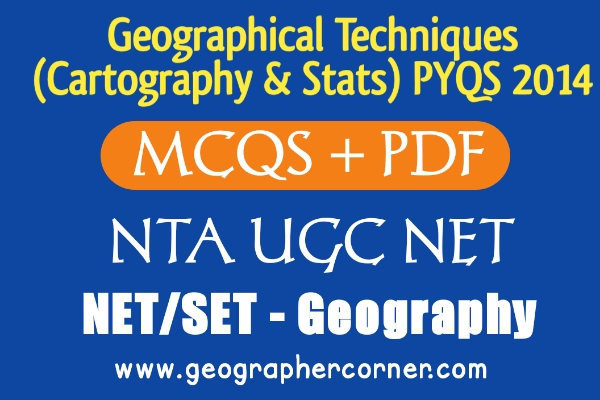# GEOGRAPHICAL TECHNIQUES PYQS JUNE & DEC 2014 NTA UGC NETGEOGRAPHICAL TECHNIQUES (CARTOGRAPHY & STATS) PYQS JUNE & DEC 2014 NTA UGC NET

GEOGRAPHICAL TECHNIQUES PYQS DEC 2014 NTA UGC NET, GEOGRAPHICAL TECHNIQUES  NTA UGC NET DEC 2014,GEOGRAPHICAL TECHNIQUES  PYQS UGC NET JUNE 2014, GEOGRAPHICAL TECHNIQUES  PYQS DEC 2014 NTA UGC NET PYQS GEOGRAPHY, GEOGRAPHICAL TECHNIQUES  PYQS UGC NET, PRACTICAL GEOGRAPHY PYQS NTA UGC NET, CARTOGRAPHY PYQS NTAUGC NET, STATS PYQS NTAUGC NET, GEOGRAPHY PYQS UGC NET,

(PDF) For download PDF file of 2014 UGC NET – go to the bottom of this post.

Q.1. Match List – I with List – II and select the correct answer from the codes given below: (UGC NET JUNE 2014)

List – I (Statistic)

(A) Standard Distance

(B) Nearest Neighbour

(C) Correlation

(D) Eigen Value

List – II (Analysis)

(i) Principal

(ii) Scatter diagram

(iii) Settlement pattern

(iv) Centrographic measure

Codes:

 A B C D (A) iv iii ii i (B) iv ii iii i (C) iii iv i ii (D) ii iii iv i

Q.2. Which of the following diagrams is used to show proportion of different land uses in an area? (UGC NET JUNE 2014)

(A) Pie

(B) Wind rose

(C) Line

(D) Flow-Chart

Q.3. Mean, median and mode coincide if the distribution of values is (UGC NET JUNE 2014)

(A) Negativety skewed

(B) Normal

(C) Positivety skewed

(D) Poisson

Q.4. The contour interval followed in SOI Toposheet with 1/50,000 scale is: (UGC NET JUNE 2014)

(A) 20 Metres

(B) 50 Metres

(C) 100 Metres

(D) 150 Metres

Q.5. Match List – I with List – II and select a correct answer using the codes given below: (UGC NET JUNE 2014)

List – I (Instruments)

(A) GPS base

(B) Pantograph

(C) Planimeter

(D) Stereoscope

List – II (Functions)

(i) Distance and area

(ii) 3-D Vision

(iii) Global location

(iv) Reduction & enlargement

Codes:

 A B C D (A) iii i iv ii (B) ii iv iii i (C) iii iv i ii (D) i ii iii iv

Q.6. The most appropriate sampling Technique to represent the heterogeneous population of a region is: (UGC NET JUNE 2014)

(A) Cluster

(B) Random stratified

(C) Systematic

(D) Purposive

##### Q.7. Flow maps are used to show the which type of distribution of following data? (UGC NET JUNE 2014)

(A) Line

(B) Area

(C) Point

(D) Volume

Q.8. Cumulative frequency distribution is shown by (UGC NET JUNE 2014)

(A) Histogram

(B) Frequency Curve

(C) Ogive

(D) Pie diagram

Q.9. Which one of the following diagrams is most suitable for depicting the distribution of urban land-use types of spatially spread urban centres? (UGC NET DEC 2014)

(A) Histogram

(B) Sphere

(C) Bar

(D) Pie

Q.10. A map with the scale of is enlarged to 5-times, which one of the following scales is correct for the enlarged map? (UGC NET DEC 2014)

(A) 1/ 250,000

(B) 1/100,000

(C) 1/10,000

(D) 1/ 2,500

Q.11. Which of the following thematic maps is combined with complex themes? Select the correct combination of complex-thematic map from the codes given below: (UGC NET DEC 2014)

(2) Forest cover

(3) Crop combination

(4) Rainfall potential

Codes:

(A) 1 and 2

(B) 2 and 3

(C) 3 and 4

(D) 2 and 4

Q.12. Which one of the following number of Parallels with one-degree interval can be drawn between the poles on a globe? (UGC NET DEC 2014)

(1) 180

(2) 178

(3) 179

(4) 181

Q.13. Which one of the following symbols does not represent a point data? (UGC NET DEC 2014)

(1) Spot heights

(2) Bench marks

(3) Contours

(4) Triangulation stations

Q.14. Which one of the following statistical techniques involves a large number of variables to be summarised into smaller dimensions? (UGC NET DEC 2014)

(A) Chi-square

(B) Correlation

(C) Factor Analysis

(D) Regression

Q.15. Which one of the following averages is not a measure of central tendency? (UGC NET DEC 2014)

(A) Harmonic mean

(B) Geographic mean centre

(C) Geometric mean

(D) Arithmetic mean

Q.16. Which one of the following circumstances Pearson’s coefficient of Correlation is used? (UGC NET DEC 2014)

(1) when the data set is positively skewed

(2) when the data set is negatively skewed

(3) when the data is normally distributed

(4) when the data is abnormally distributed